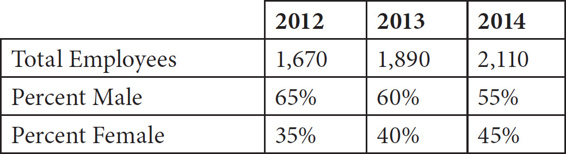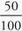# SAT Math Multiple Choice Question 133: Answer and Explanation

### Test Information

Question: 133

13. The chart below shows data about the number of employees at Cuda Cola, a popular beverage company.Assuming the employee total grows at the same rate each year, and male and female percentages continue to decrease and increase by 5%, respectively, approximately how many male employees will work at Cuda Cola in 2015 ?

• A. 1515
• B. 1398
• C. 1282
• D. 1165

D The question states that the number of employees increases at the same rate per year, so you can determine this numerical increase by subtracting one year's total from the next year's total. Subtracting the 2013 total from the 2014 total gives 2,110 - 1,890 = 220. To find the total in 2015, add 220 to the 2014 total: 2,110 + 220 = 2,330. The question also states that the male percentages continue to decrease at the same rate, which, based on the data in the table, is 5% per year. The percent male in 2014 was 55%, so the percent male in 2015 will be 50%. 50% of 2,330 can be expressed as or(2330) = 1,165, which is (D).